Clustering with PCA and Kmeans

In this post I will use the census API discussed in the last post to cluster the Allegheny County census tracts using PCA and k-means.

Setup

library(tidyverse)
library(tidycensus)
library(tigris)
library(sf)
library(broom)
library(ggfortify)
library(viridis)
library(janitor)
library(scales)
library(ggthemes)

options(tigris_use_cache = TRUE)

theme_set(theme_minimal())
census_api_key(my_census_key)

census_vars <- load_variables(2010, "sf1", cache = TRUE)

Census tracts are small geographic areas analogous to local neighborhoods. This is a map of all the tracts in Allegheny County, for reference: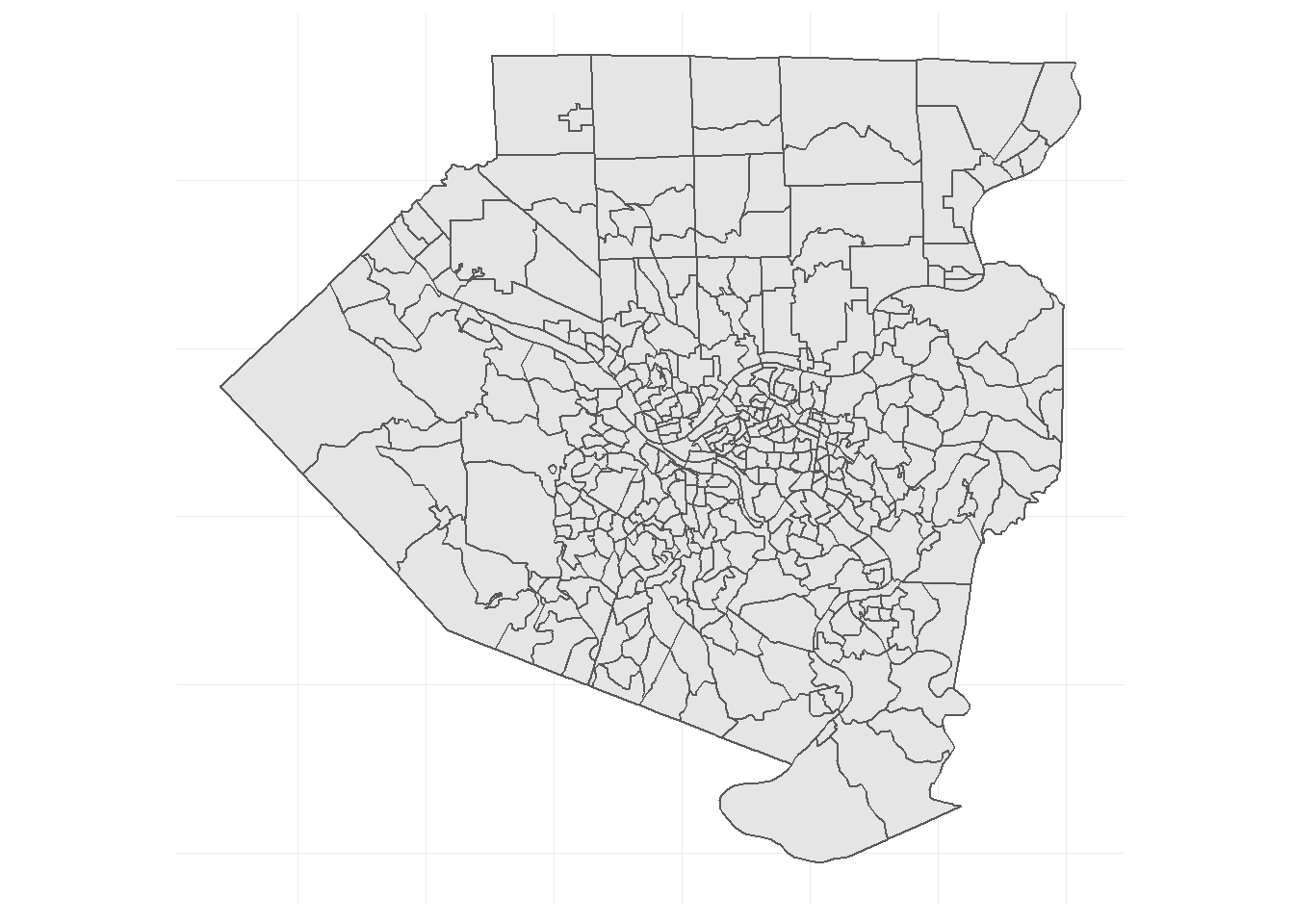This code downloads data about the ethnicities resident in the tracts and calculates them as a % of the tract population.

vars_demo <- c(white = "P0050003",
black = "P0050004",
asian = "P0050006",
hispanic = "P0040003")
#age vars men and women
#P0120003:P0120049

get_decennial(geography = "tract",
variables = vars_demo,
state = "PA",
county = "Allegheny",
geometry = FALSE,
summary_var = "P0010001") %>%
arrange(GEOID) %>%
mutate(value = value / summary_value) %>%
select(-summary_value) %>%
rename_at(vars("white", "black", "asian", "hispanic"), funs(str_c("pct_", .))) -> allegheny_demographics

allegheny_demographics <- replace(allegheny_demographics, is.na(allegheny_demographics), 0)

This code downloads information about the housing stock in each tract, specifically what % of housing units are owned outright, owned with a loan, or rented.

vars_housing <- c(units_owned_loan = "H0110002",
units_owned_entire = "H0110003",
units_rented = "H0110004")

get_decennial(geography = "tract",
variables = vars_housing,
state = "PA",
county = "Allegheny",
geometry = FALSE,
summary_var = "H0110001") %>%
arrange(GEOID) %>%
mutate(value = value / summary_value) %>%
select(-summary_value) %>%
rename_at(vars("units_owned_entire", "units_owned_loan", "units_rented"), funs(str_c("pct_", .))) -> allegheny_housing

allegheny_housing <- replace(allegheny_housing, is.na(allegheny_housing), 0)

This code requests the total population of each tract.

#originally I used age-sex variables, but they were not useful
vars_age_total <- census_vars %>%
filter(concept == "P12. Sex By Age ",
label != "Total population",
label != "Male:")

get_decennial(geography = "tract",
variables = vars_age_total$name, state = "PA", county = "Allegheny", geometry = FALSE, summary_var = "P0120001") %>% rename(var_id = variable) %>% mutate(value = value / summary_value) %>% spread(var_id, value) -> allegheny_age_sex colnames(allegheny_age_sex) <- c("GEOID", "NAME", "summary_value", vars_age_total$label)

allegheny_age_sex %>%
clean_names() %>%
rename(GEOID = geoid,
NAME = name,
total_population = summary_value) -> allegheny_age_sex

allegheny_age_sex <- replace(allegheny_age_sex, is.na(allegheny_age_sex), 0)

allegheny_age_sex %>%
select(GEOID, NAME, total_population) -> allegheny_age_sex

This code requests the geometry of each tract that I will use to map them later.

get_decennial(geography = "tract",
variables = vars_housing,
state = "PA",
county = "Allegheny",
geometry = TRUE) %>%
select(-c(variable, value)) -> allegheny_geo

This joins the 4 dataframes together.

allegheny_geo %>%
left_join(allegheny_housing) %>%
left_join(allegheny_demographics) %>%
left_join(allegheny_age_sex) %>%
mutate(id = str_c(GEOID, NAME, sep = " | ")) %>%
distinct() -> allegheny

Exploratory graph

This graph compares the percent of white residents to the remaining variables in the data. pct_white is on the x axis of each of the smaller charts. Note that each chart’s Y axis has its own scale. It is already obvious that pct_white and pct_black are negatively correlated with each other.

allegheny %>%
st_set_geometry(NULL) %>%
select(contains("pct")) %>%
gather(variable, value, -pct_white) %>%
ggplot(aes(pct_white, value)) +
geom_point(alpha = .5) +
geom_smooth() +
facet_wrap(~variable, scales = "free", nrow = 3, strip.position="left") +
scale_x_continuous(label = percent) +
scale_y_continuous(label = percent) +
labs(x = NULL) +
theme_bw() +
theme(strip.placement = "outside")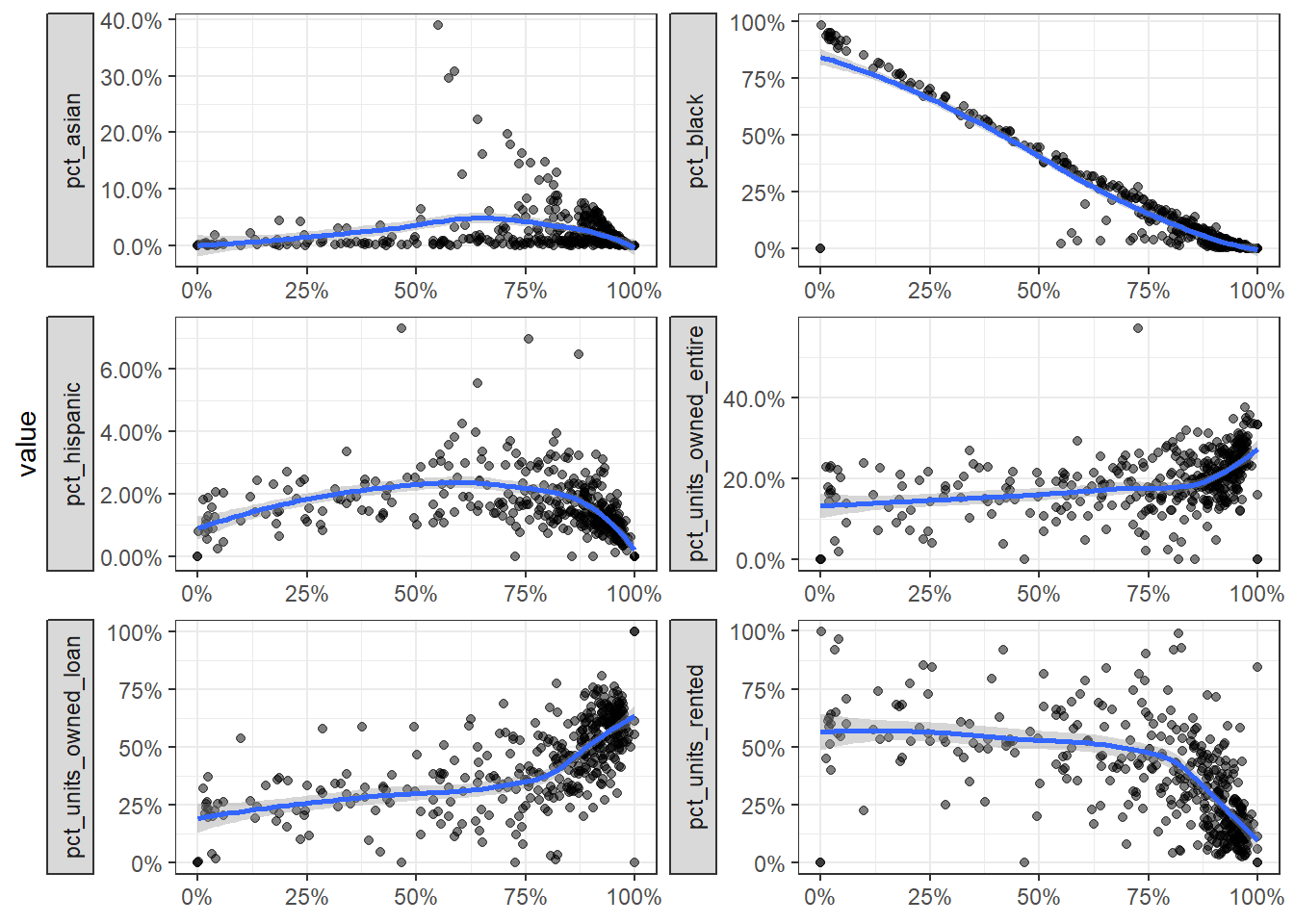This code plots the total population against the other variables:

allegheny %>%
st_set_geometry(NULL) %>%
select(contains("pct"), total_population) %>%
gather(variable, value, -total_population) %>%
ggplot(aes(total_population, value)) +
geom_point(alpha = .5) +
geom_smooth() +
facet_wrap(~variable, scales = "free", nrow = 3, strip.position="left") +
scale_x_continuous(label = comma) +
scale_y_continuous(label = percent) +
theme_bw() +
theme(strip.placement = "outside")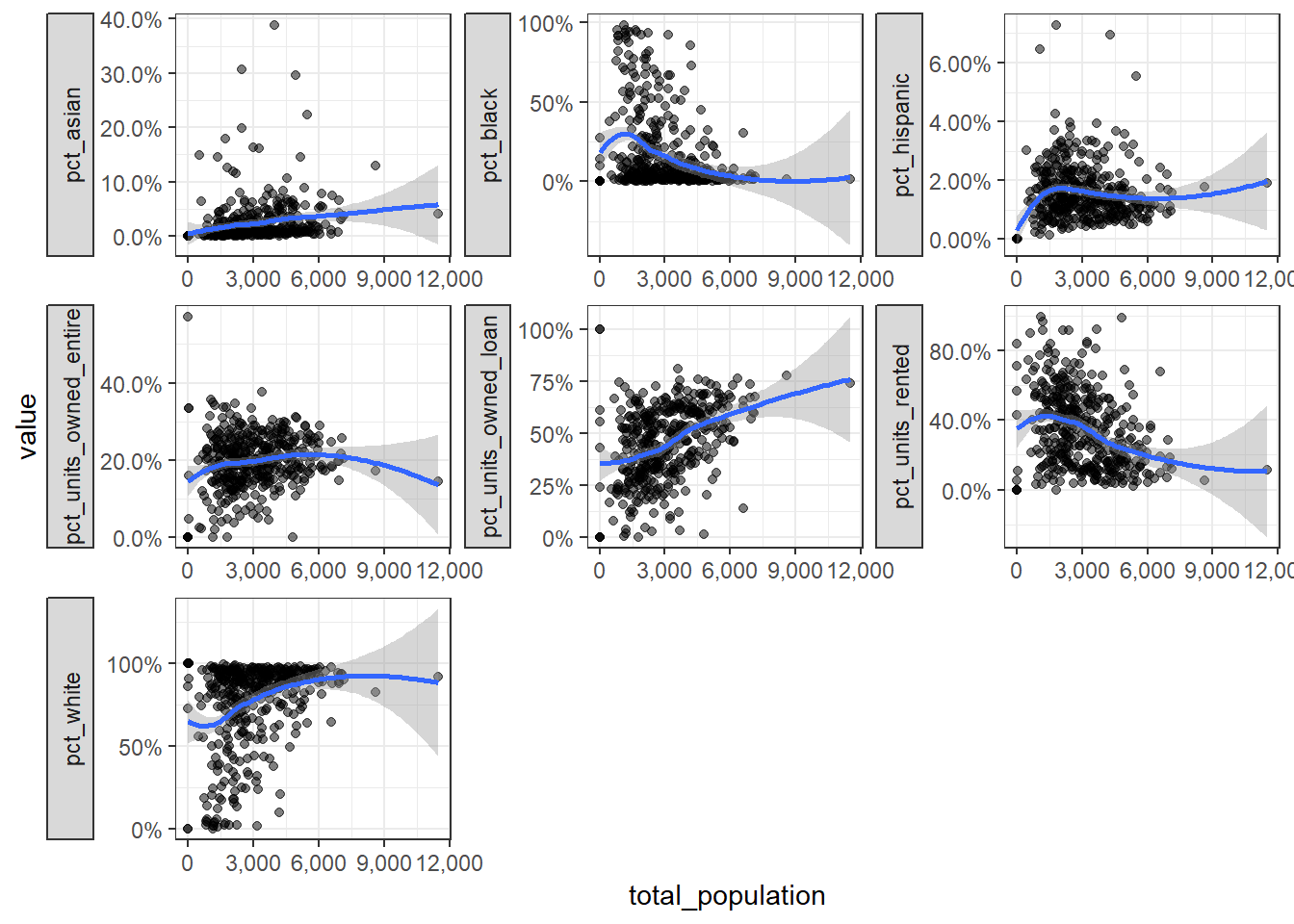Prepare for PCA

This code prepares the data for PCA:

allegheny %>%
select(-c(id, GEOID)) %>%
st_set_geometry(NULL) %>%
remove_rownames() %>%
column_to_rownames(var = "NAME") -> allegheny_pca

allegheny_pca %>%
prcomp(scale = TRUE) -> pc

This shows how the PCs explain the variance in the data. As explained earlier, the first few PCs explain most of the variance in the data.

pc %>%
tidy("pcs") %>%
select(-std.dev) %>%
gather(measure, value, -PC) %>%
mutate(measure = case_when(measure == "percent" ~ "Percent",
measure == "cumulative" ~ "Cumulative")) %>%
ggplot(aes(PC, value)) +
geom_line() +
geom_point() +
facet_wrap(~measure) +
labs(title = "Variance explained by each principal component",
x = "Principal Component",
y = NULL) +
scale_x_continuous(breaks = 1:8) +
scale_y_continuous(label = percent) +
theme_bw()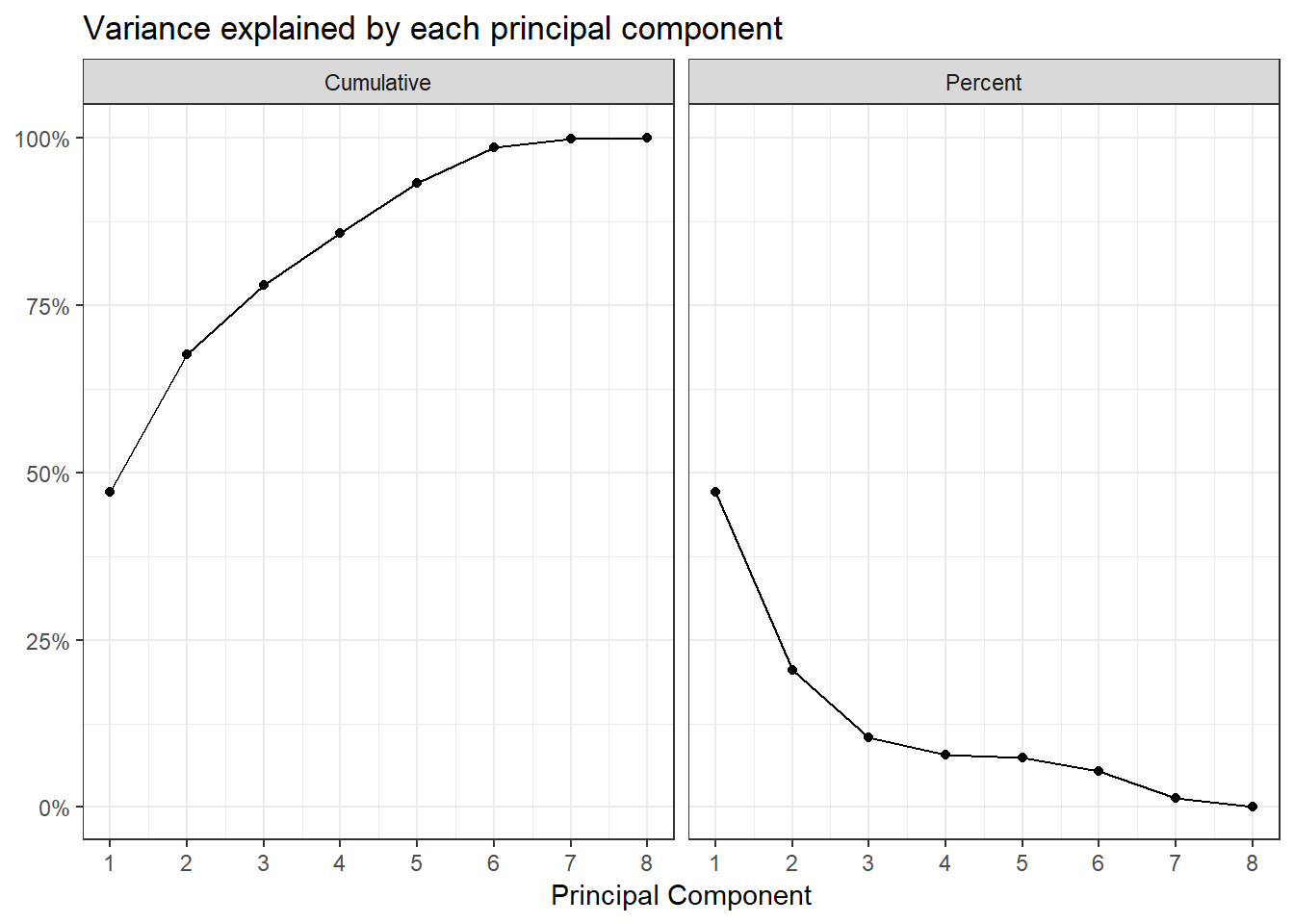This shows how the PCA function rearranged the data to maximize the variance in the first few PCs. PC1 is largely defined by the percent of a tract that is white or black, the percent of housing units that are owned, and the total population of the tract. The “pct_white” and “pct_black” arrows point in opposite directions, which reflects Pittsburgh’s status as a segregated city.

PC2 explains less of the variance, and is influenced by the percent of a tract that is hispanic, asian, or black.

allegheny %>%
select(-c(id, GEOID)) %>%
st_set_geometry(NULL) %>%
nest() %>%
mutate(pca = map(data, ~ prcomp(.x %>% select(-NAME),
center = TRUE, scale = TRUE)),
pca_aug = map2(pca, data, ~augment(.x, data = .y))) -> allegheny_pca2

allegheny_pca2 %>%
mutate(
pca_graph = map2(
.x = pca,
.y = data,
data = .y) +
theme_bw() +
labs(x = "Principal Component 1",
y = "Principal Component 2",
title = "First two principal components of PCA on Allegheny County Census data")
)
) %>%
pull(pca_graph)
## []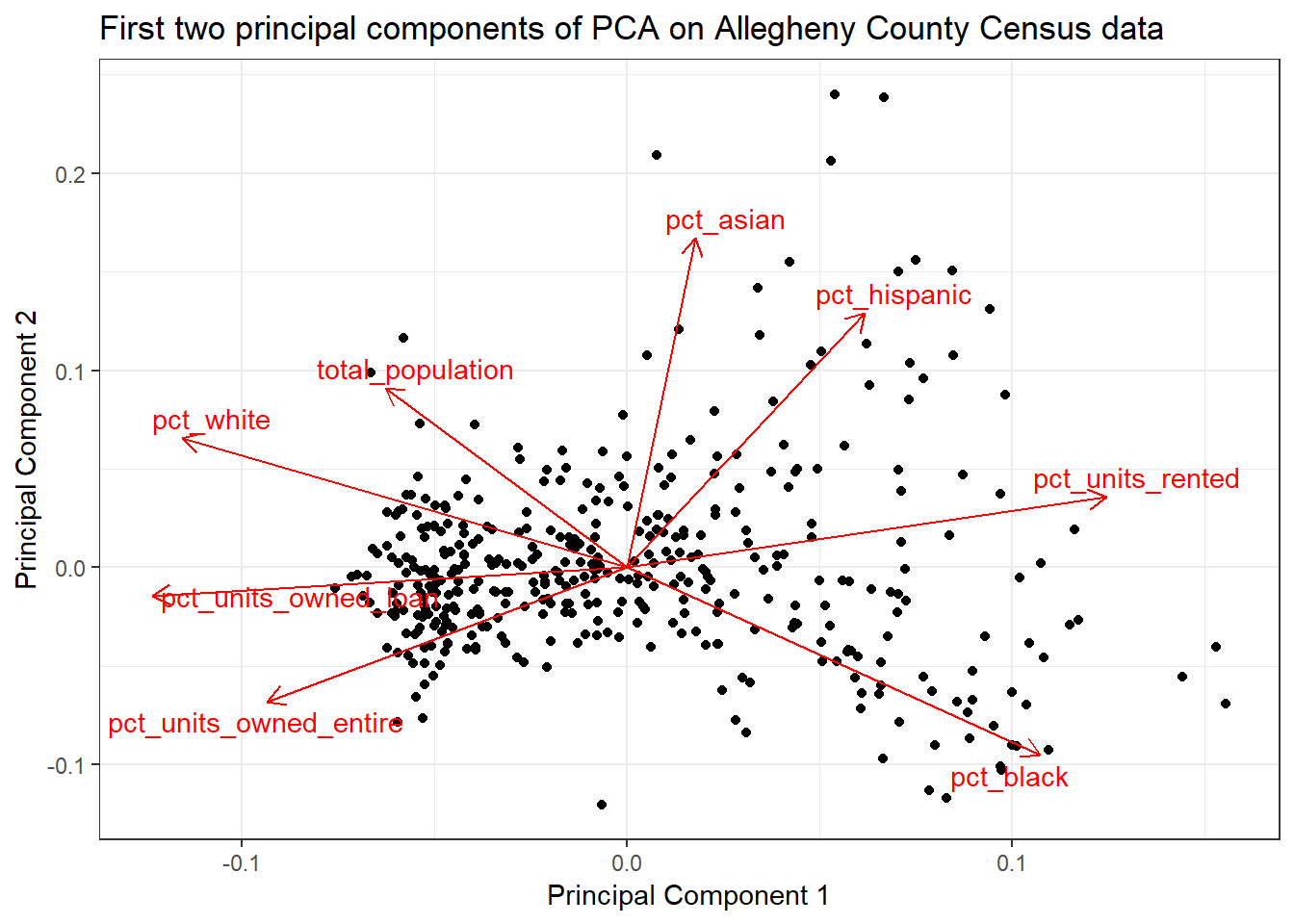This code maps the first two PCs to the tracts.

df_au %>%
rename(NAME = .rownames) %>%
gather(variable, value, -c(NAME)) -> df_au_long

st_erase <- function(x, y) {
st_difference(x, st_union(st_combine(y)))
}

allegheny_water <- area_water("PA", "Allegheny", class = "sf")

allegheny_geo <- st_erase(allegheny_geo, allegheny_water)

allegheny_geo %>%
left_join(df_au %>% rename(NAME = .rownames)) %>%
gather(pc, pc_value, contains(".fitted")) %>%
mutate(pc = str_replace(pc, ".fitted", "")) -> allegheny_pca_map

allegheny_pca_map %>%
filter(pc %in% c("PC1", "PC2")) %>%
ggplot(aes(fill = pc_value, color = pc_value)) +
geom_sf() +
facet_wrap(~pc) +
coord_sf(crs = 26911) +
scale_fill_viridis("Principal component value", option = "magma") +
scale_color_viridis("Principal component value", option = "magma") +
labs(title = "Allegheny County",
subtitle = "American Community Survey") +
theme(axis.text = element_blank())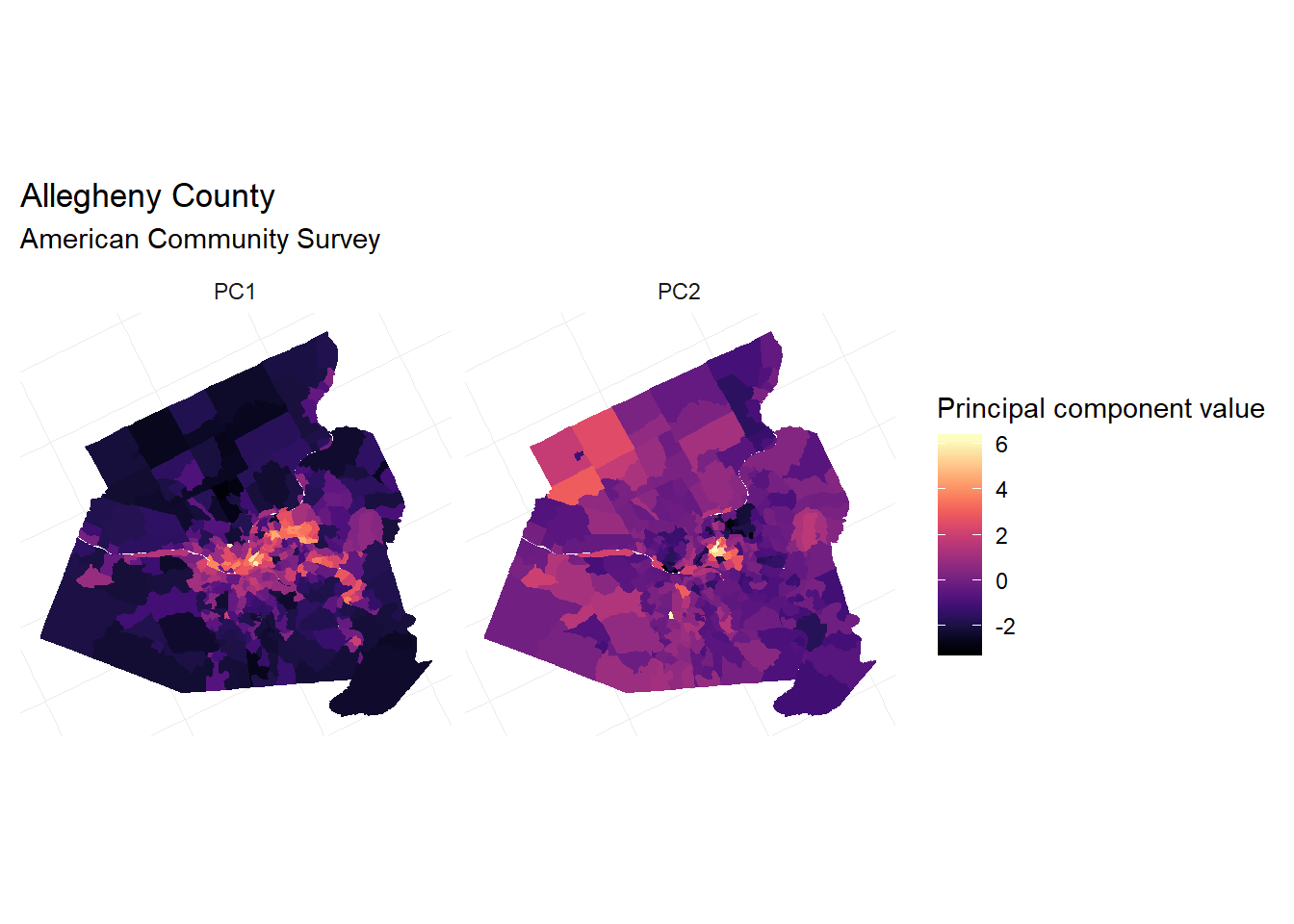Clustering with k-means

Next I will use k-means to cluster the PC data.

df_au_long %>%
filter(str_detect(variable, "PC")) %>%
select(-NAME) -> allegheny_kmeans

This code clusters the data using 1 to 9 clusters.

kclusts <- tibble(k = 1:9) %>%
mutate(
kclust = map(k, ~kmeans(allegheny_kmeans, .x)),
tidied = map(kclust, tidy),
glanced = map(kclust, glance),
augmented = map(kclust, augment, allegheny_kmeans)
)
clusters <- kclusts %>%
unnest(tidied)

assignments <- kclusts %>%
unnest(augmented)

clusterings <- kclusts %>%
unnest(glanced, .drop = TRUE)

Based on this “elbow chart”, the optimum number of clusters is most likely 2.

ggplot(clusterings, aes(k, tot.withinss)) +
geom_line() +
geom_point() +
geom_vline(xintercept = 2, linetype = 2) +
scale_x_continuous(breaks = 1:9) +
labs(x = "Number of clusters",
y = "Between-cluster sum of squares")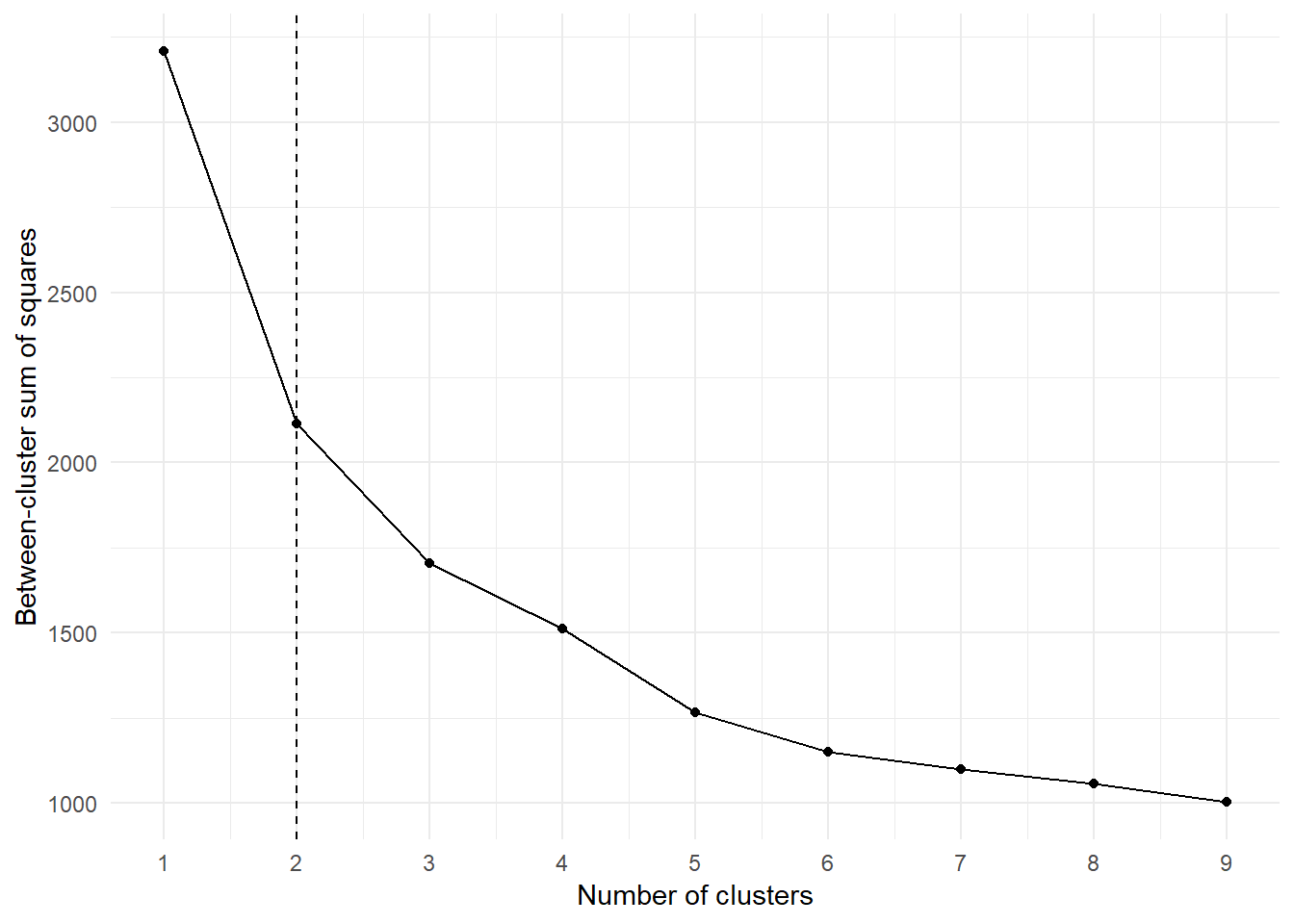We can visualize how the data would look if it were assigned to a different number of clusters. Clearly the clustering algorithm experiences diminishing returns after 2 or 3 clusters.

ggplot(assignments, aes(.fittedPC1, .fittedPC2)) +
geom_point(aes(color = .cluster), alpha = .7) +
facet_wrap(~ str_c(k, " cluster(s)")) +
scale_color_discrete("Cluster") +
labs(x = "PC1",
y = "PC2") +
theme_bw()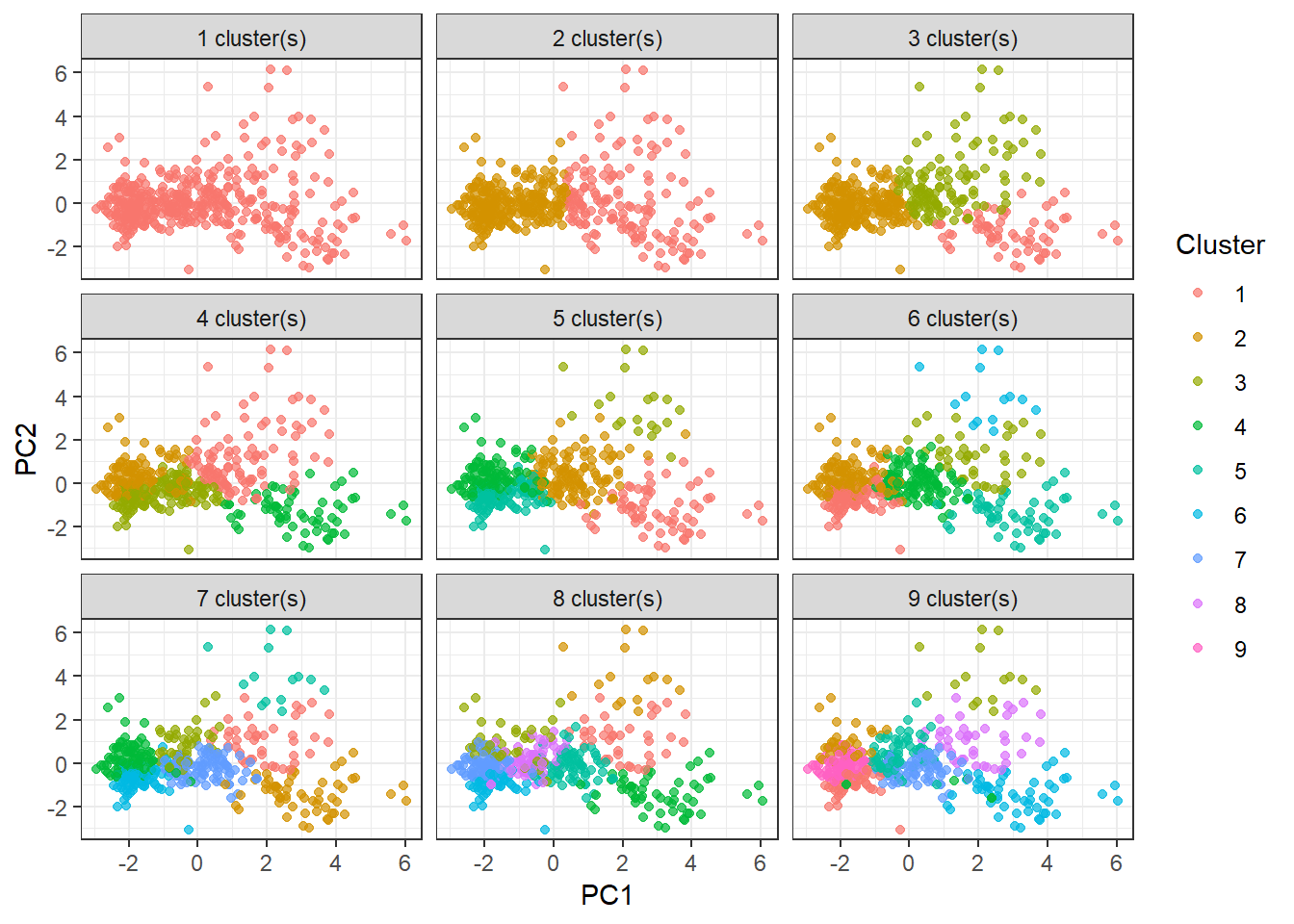This code divides the data into 2 clusters and maps the clusters onto the tract map.

df_au_long %>%
filter(str_detect(variable, "PC")) %>%
select(-NAME) -> allegheny_kmeans

kclust <- kmeans(allegheny_kmeans, centers = 2)

kclust %>%
augment(df_au_long %>%
filter(str_detect(variable, ".fitted")) %>%

get_decennial(geography = "tract",
variables = vars_housing,
state = "PA",
county = "Allegheny",
geometry = TRUE) %>%
select(-c(variable, value)) -> allegheny_geo

allegheny_geo %>%
left_join(allegheny_kmeans) -> allegheny
st_erase <- function(x, y) {
st_difference(x, st_union(st_combine(y)))
}

allegheny_water <- area_water("PA", "Allegheny", class = "sf")

allegheny <- st_erase(allegheny, allegheny_water)

allegheny %>%
ggplot(aes(fill = .cluster, color = .cluster)) +
geom_sf(color = "grey") +
scale_fill_viridis("Cluster", discrete = TRUE, direction = 1) +
scale_color_viridis("Cluster", discrete = TRUE, direction = 1) +
theme(axis.text = element_blank())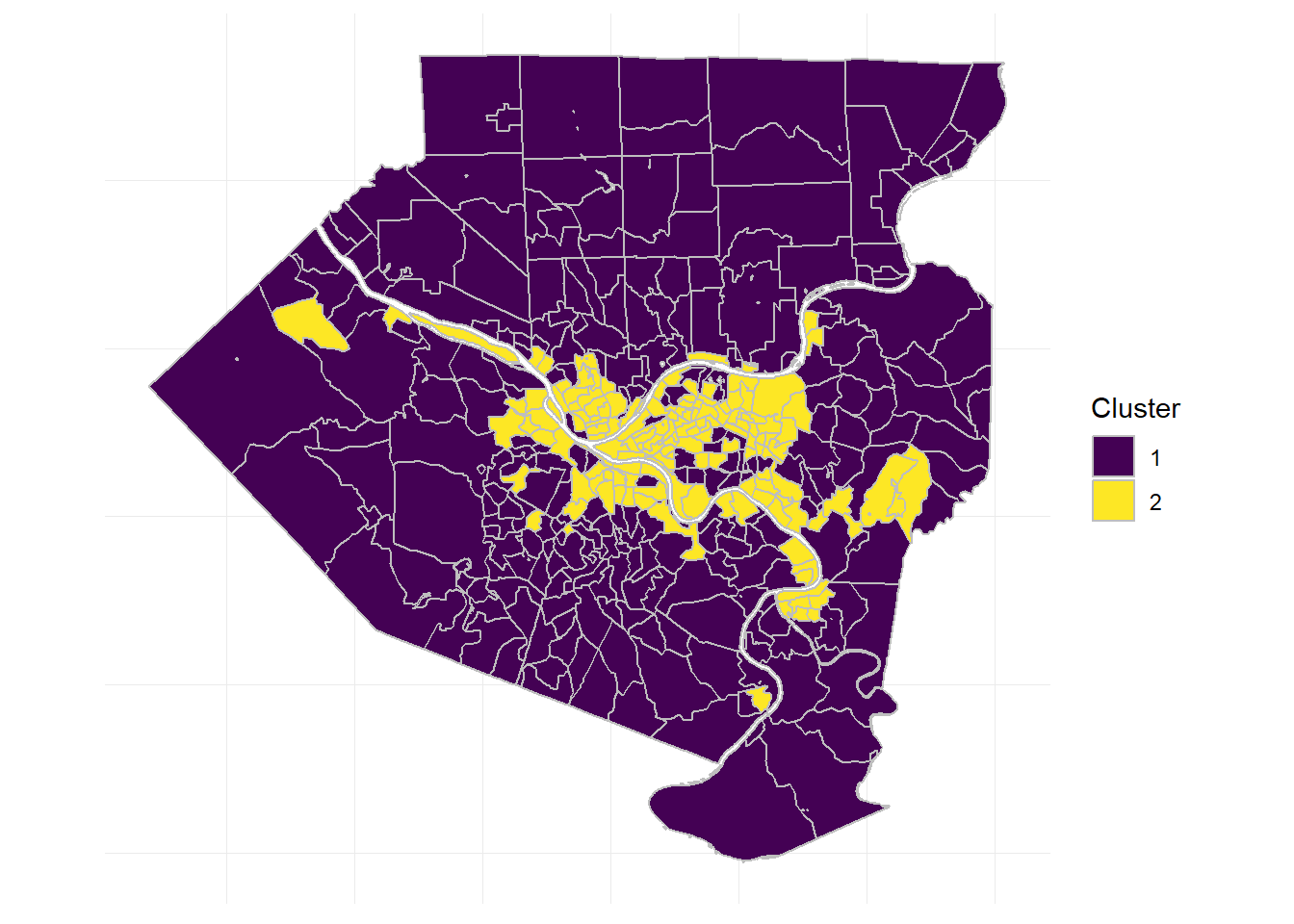The second cluster largely follows the city limits, but excludes areas such as Mount Washington, Squirrel Hill, and Shadyside. It also includes a few areas outside of the city like Duquesne and McKeesport.